# Second-order curve

(diff) ← Older revision | Latest revision (diff) | Newer revision → (diff)

A plane curve whose rectangular Cartesian coordinates satisfy an algebraic equation of the second degree:(*)

Equation (*) need not define a real geometrical form, but to preserve generality in such situations one says that it defines an imaginary second-order curve. Depending on the values of the coefficients of the equation (*) it can be transformed by parallel displacement and rotation of the coordinate system through some angle to one of the 9 canonical forms given below, to each of which there corresponds a definite class of curves. Namely,

non-degenerate curves:, ellipses (cf. Ellipse);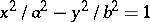, hyperbolas (cf. Hyperbola);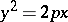, parabolas (cf. Parabola);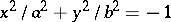, imaginary ellipses;

degenerate curves: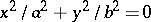, pairs of imaginary intersecting lines;, pairs of real intersecting lines;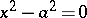, pairs of real parallel lines;, pairs of imaginary parallel lines;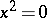, a pair of coincident real lines.

A second-order curve that has a unique centre of symmetry (the centre of the second-order curve) is called a central curve. The coordinates of the centre of a second-order curve are determined by the solution of the system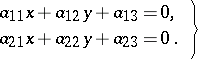A second-order curve without a centre of symmetry or with an indefinite centre is called a non-central curve.

Investigations on the form of a second-order curve can be carried out without reducing the general equation to canonical form. This is done by considering the values of the so-called fundamental invariants of a second-order curve, that is, the following expressions in the coefficients of the equation (*), the values of which are not changed under a parallel displacement and a rotation of the coordinate system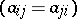:and the semi-invariantwhich is an invariant with respect to rotation of the coordinate system (see the Table below).

Many important properties of second-order curves can be studied by means of the characteristic quadratic formcorresponding to equation (*). In particular, a non-degenerate second-order curve turns out to be an ellipse, an imaginary ellipse, a hyperbola, or a parabola, depending on whether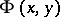is a positive-definite, a negative-definite, an indefinite, or a semi-definite quadratic form. This can be established from the roots of its characteristic equation: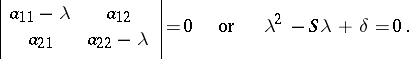The three fundamental invariants,anddetermine a second-order curve (excluding the case of parallel lines) up to a motion of the Euclidean plane: If the corresponding invariants,andof two curves are equal, then these curves can be made to coincide by a motion. In other words, these curves are equivalent with respect to the group of motions of the plane (metrically equivalent).

There is a classification of second-order curves from the point of view of other groups of transformations. Thus, with respect to the more general (than the group of motions) group of affine transformations any two curves defined by equations of the same canonical form are equivalent. For example, two similar second-order curves are regarded as equivalent. The connections between different affine classes of second-order curves make it possible to establish a classification from the point of view of projective geometry, in which elements at infinity do not play a special role. The real non-degenerate second-order curves, ellipses, hyperbolas, and parabolas, form one projective class — the class of real oval curves (ovals, cf. Oval). A real oval curve is an ellipse, hyperbola or parabola, depending on how it is situated with respect to the line at infinity; an ellipse intersects the line at infinity in two imaginary points, a hyperbola intersects it in two real points and a parabola touches it; there are projective transformations that take these curves into one another. There are 5 projective equivalence classes of second-order curves. Namely,

non-degenerate curves (are the homogeneous coordinates):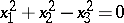, a real oval;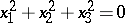, an imaginary oval;

degenerate curves: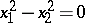, a pair of real lines;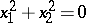, a pair of imaginary lines;, a pair of coincident lines.

Apart from the analytic method of defining second-order curves (specifying the equation) there are other methods. For example, an ellipse, hyperbola and parabola can be obtained as a section of a conical surface by a plane (see Conic sections).'

<tbody> </tbody>
 Non-degenerate curves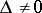Degenerate curvesCentral curves (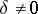)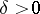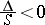Real ellipses Pairs of intersecting straight lines (a real point)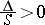Imaginary ellipses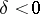Hyperbolas Pairs of real non-intersecting straight lines Non-central curves ()Parabolas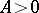Pairs of imaginary parallel linesPairs of real parallel lines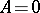Pairs of coincident parallel lines
  P.S. Aleksandrov, "Lectures on analytical geometry" , Moscow (1968) (In Russian)  N.V. Efimov, "A short course of analytical geometry" , Moscow (1967) (In Russian)